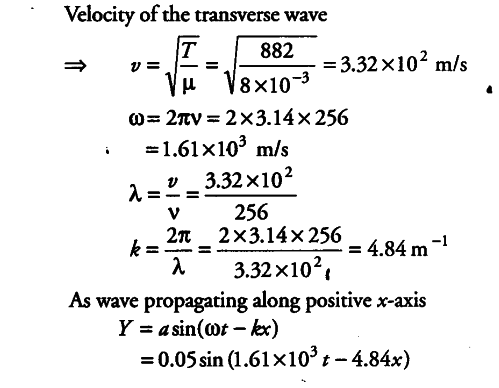# One end of a long string of linear mass density

One end of a long string of linear mass density 8.0xl0_3kg${{m}^{-1}}$ is connected to an electrically driven tuning fork of frequency 256 Hz. The other end passes over a pulley and is tied to a pan containing a mass of 90 kg. The pulley end absorbs all the incoming energy so that reflected waves at the end have negligible amplitude. At t = 0, the left, and (fork end) of the string x = 0 has zero transverse displacement (y = 0) and is moving along positive y-direction. The amplitude of the wave is 5.0 cm. Write down the transverse displacement y as function of x and t that describes the wave on the string.

v = 256 Hz, T = 90 x 9.8 = 882 N

\mu = m/L = 8.0 x {{10}^{-3}} Kg${{m}^{-1}}$